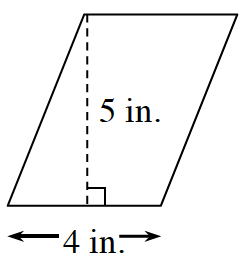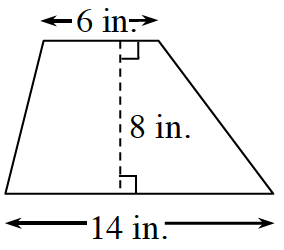### Home > CC1MN > Chapter 6 > Lesson 6.3.4 > Problem6-101

6-101.

Choose any strategy to find the area of each shape below. Use the information in the Math Notes boxes for help. Assume that the shape in part (a) is a parallelogram and that the shape in part (b) is a trapezoid.

1.1.• Form a rectangle using the left portion of the parallelogram. Then find the area of the rectangle.

• When the trapezoid is copied and rotated, a parallelogram can be formed.

Form a parallelogram using the rotated trapezoid.

Now find the area of the parallelogram.

Use this area to find the area of the original trapezoid.